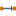#### Versions

You are viewing the RapidMiner Studio documentation for version 8.2 - Check here for latest version

#Similarity to Data (RapidMiner Studio Core)

## Synopsis

This operator calculates an ExampleSet from the given similarity measure.

## Description

The Similarity to Data operator calculates an ExampleSet from the given SimilarityMeasure Object. The ExampleSet can be in form of a long table or a matrix. This behavior can be controlled by the table type parameter. A similarity measure object contains the calculated similarity between each example of an ExampleSet with every other example of the same ExampleSet. Operators like the Data to Similarity operator can generate a similarity measure object.

## Input

•similarity (Similarity Measure)

This input port expects a similarity measure object. A similarity measure object contains the calculated similarity between each example of an ExampleSet with every other example of the same ExampleSet. The Data to Similarity operator can generate a similarity measure object.

•example set (Data Table)

This input port expects an ExampleSet. It is the output of the Data to Similarity operator in the attached Example Process. The output of other operators can also be used as input.

## Output

•example set (Data Table)

An ExampleSet is calculated from the given similarity measure and it is returned from this port.

## Parameters

• table_typeThis parameter indicates if the resulting table should have a matrix format or a long table format. Range: selection

## Tutorial Processes

### Introduction to the Similarity to Data operator

The 'Golf' data set is loaded using the Retrieve operator. A breakpoint is inserted here so that you can have a look the ExampleSet. You can see that the ExampleSet has 14 examples. The Data to Similarity operator is applied on it to compute the similarity of examples. As there are 14 examples in the given ExampleSet, there will be 91 (i.e. (14)(14-1)/2) similarity comparisons in the resultant similarity measure object. A breakpoint is inserted here so that you can have a look at this SimilarityMeasure Object. The Similarity to Data operator is applied on this SimilarityMeasure Object to calculate an ExampleSet. The table type parameter is set to 'matrix', therefore the resultant ExampleSet is in the form of a matrix. It can be seen in the Results Workspace.